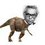# Why are we caring so much about the existence of such a tiny Point P?

Consider a quadrilateral $ABCD$ . Find the necessary and sufficient condition with proof so that there exists a point $P$ in the interior of $ABCD$ such that $A(PAB)=A(PBC)= A(PCD)= A(PDA)$.

$\text{A( ) represents Area}$

Nice solutions are always welcome!Note by Nihar Mahajan
6 years, 2 months ago

This discussion board is a place to discuss our Daily Challenges and the math and science related to those challenges. Explanations are more than just a solution — they should explain the steps and thinking strategies that you used to obtain the solution. Comments should further the discussion of math and science.

When posting on Brilliant:

• Use the emojis to react to an explanation, whether you're congratulating a job well done , or just really confused .
• Ask specific questions about the challenge or the steps in somebody's explanation. Well-posed questions can add a lot to the discussion, but posting "I don't understand!" doesn't help anyone.
• Try to contribute something new to the discussion, whether it is an extension, generalization or other idea related to the challenge.

MarkdownAppears as
*italics* or _italics_ italics
**bold** or __bold__ bold
- bulleted- list
• bulleted
• list
1. numbered2. list
1. numbered
2. list
Note: you must add a full line of space before and after lists for them to show up correctly
paragraph 1paragraph 2

paragraph 1

paragraph 2

[example link](https://brilliant.org)example link
> This is a quote
This is a quote
    # I indented these lines
# 4 spaces, and now they show
# up as a code block.

print "hello world"
# I indented these lines
# 4 spaces, and now they show
# up as a code block.

print "hello world"
MathAppears as
Remember to wrap math in $$ ... $$ or $ ... $ to ensure proper formatting.
2 \times 3 $2 \times 3$
2^{34} $2^{34}$
a_{i-1} $a_{i-1}$
\frac{2}{3} $\frac{2}{3}$
\sqrt{2} $\sqrt{2}$
\sum_{i=1}^3 $\sum_{i=1}^3$
\sin \theta $\sin \theta$
\boxed{123} $\boxed{123}$

Sort by:

Such a point $P$ exists if and only if one diagonal bisects the area of the quadrilateral.

- 6 years, 2 months ago

Can you please provide the proof sir ?

- 6 years, 2 months ago

Sir, thanks for your answer.But it may be more beneficial for us if you post a nice proof.

- 6 years, 2 months ago

Now that you know the right condition, you should try proving it.

- 6 years, 2 months ago

Sir, actually this problem is from the homework of our class, and our sir has a different condition- P exists iff one diagonal bisects the other.

- 6 years, 2 months ago

Yeah , thats why I posted this as a note.

- 6 years, 2 months ago

Did you get a proof

- 6 years, 2 months ago

I got the proof for the condition- P exists iff one diagonal bisects the other.I did not get proof for the condition that Jon Hausmann has posted.

- 6 years, 2 months ago

yes,same

- 6 years, 2 months ago

In a quadrilateral, the conditions "one diagonal bisects the area" and "one diagonal bisects the other diagonal" are equivalent.

- 6 years, 2 months ago

Oh yes, thank you for pointing that out to me

- 6 years, 2 months ago

- 6 years, 2 months ago

Nihar, Tujhe pata hai bhai ki Mujhe Geometry nahi aati :3 Jo Bhi Ho. Thanks For @Mentioning Me :D

- 6 years, 2 months ago

I mentioned you so that you will have something interesting in geometry. ;)

- 6 years, 2 months ago

Yeah, Thanks! :) :D :)

- 6 years, 2 months ago

me , @Kalash Verma @Harsh Shrivastava @CH Nikhil are in need of a nice solution.Thanks!

- 6 years, 2 months ago